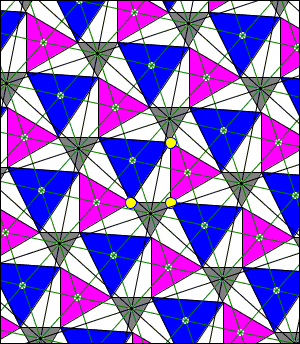# Napoleon's Theorem by Plane Tessellation

We already have several proofs of Napoleon's Theorem and its generalizations. Here is an illustration of another one that is somewhat akin to the second proof by Scott Brodie but (to me is) more revealing.

### This applet requires Sun's Java VM 2 which your browser may perceive as a popup. Which it is not. If you want to see the applet work, visit Sun's website at https://www.java.com/en/download/index.jsp, download and install Java VM and enjoy the applet.

 What if applet does not run?The given triangle is the one at whose vertices I placed yellow dots. (These are draggable so that you must be able to modify the triangle.) It appears that the triangle and the attached Napoleon's triangles form a starting configuration for a simple tessellation of the plane. Napoleon's triangles have different backgrounds. To clarify the argument, pick two colors and erase all other triangles. Then it becomes practically obvious that the centers of triangles of a single color form a hexagonal lattice (black lines).

Centers of all other triangles lie at the centers of the lattice triangles. In the diagram, all such points are connected by greenish lines. The greenish lines form a finer lattice (a lattice with smaller base region.) Centers of all Napoleon's triangles lie at the nodes of the latter lattice. This proves the theorem.

Note that the three lattices generated by triangles of different colors form yet another hexagonal lattice, a super-lattice of that formed by the centers of Napoleon's triangles.

Various plane tessellations are discussed in a recent book Dissections: Plane & Fancy (Cambridge University Press, 1997) by Greg Frederickson from Purdue University. Although Napoleon's theorem is not mentioned in the book, you can get a sense how this approach applies to other problems. For another example you are referred to the proof of Pythagoras' Theprem by K.O.Friedrichs.

## Reference

1. G. N. Frederickson, Dissections: Plane & Fancy, Cambridge University Press, 1997
2. P.Scott, Some Recent Discoveries in Elementary Geometry, The Math Gazette, 1998, pp 391-397
3. J.F.Rigby, Napoleon Revisited, J of Geometry, 33 (1988) pp 129-146